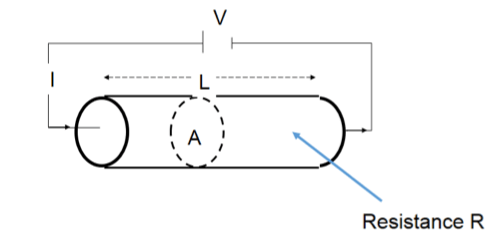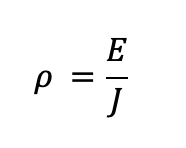# What is Electrical Resistivity?

Electrical resistivity is a property unique to every material, which is essential to understand before creating and designing electrical and electronic systems. Knowledge of how materials differ in resistivity provides information for selecting appropriate materials used for building motors, electrical circuits, dielectrics, resistive heating, and superconducting applications.

## What is the electrical resistivity of a material?

Electrical resistivity, represented by the Greek letter ρ (rho), is a measure of the resistance of a specific material of a given size, to the electrical current conduction that flows through it. It is also referred to as the specific electrical resistance or volume resistivity . The SI unit of electrical resistivity is expressed in ohm-metres (Ωm). It is also found in units of (μΩcm). Insulators have high values of electrical resistivity in the range of 1010 Ωm or more, whereas metal conductors have very small resistivity values in the range of  10-8 Ωm.

## What is the formula of electrical resistivity?

The electrical resistivity (ρ) of a solid object is determined by passing an electric current through a specimen and then measuring the resultant voltage drop over a certain length. It is expressed by the relationship between the electrical field inside the material and the flowing electric current.

The fundamental relationship between a material’s opposition to electron flow is represented by Ohm’s law :

where,

V = I . R

V is the applied voltage, (volts: V)

I is the electrica

l current flow (amperes: A)

R is the resistance of the material (ohms: Ω)Image 1 Source for reference - https://www.subsurfaceinsights.com/images/ohmslaw.png

By dividing the voltage by the specimen length L resulting in an electric field E and the current by the cross-sectional area A resulting in a current density J, electrical resistivity can be described as an intrinsic material property, as follows:ρ is the electrical resistivity of the material (ohm-metre: Ωm)

E is the magnitude of the electric field in the material (volt/metre: V/m)

J is the magnitude of the electric current density in the material (ampere/sq.m: A/m2)

For an ideal resistor or conductor that has a uniform cross-section, physical composition, and uniform current flow, the electrical resistivity formula can be written as:where,

R is the electrical resistance of a uniform specimen (ohms: Ω)

A is the cross-sectional area of the specimen (square metres: m2)

L is the length of the specimen (metres: m) 

Conductivity, in contrast to resistivity, is a material property that relates to the ease at which electric current could flow in a material. It is the reciprocal of resistivity and is given by 1/ρ with a unit of Siemens/metre (S/m). A superconductor has virtually zero ohmic resistance and infinite conductance .

## Resistivity vs Resistance

Resistivity and resistance must not be confused with each other. Resistivity is a material property with an intrinsic value just like thermal conductivity, while resistance is influenced by the shape, geometry, and resistivity. Consider two rod samples of a conducting material with the same composition and length but of different diameters. The resistivity is expected to be the same in both, yet the one with the smaller cross-sectional area will have a relatively greater resistance. If, however, the diameters were the same and the length of one rod is increased, the longer rod will have a greater resistance. Therefore, we could say that for a conductor, resistance is proportional to its length (R ∝ L) .

## Resistivity and temperature

The electrical resistivity is dependent on temperature and, for most materials, the tabular values are normally given at room temperature (20°C). Metallic conductors usually have increasing resistivity in proportion to temperature, whereas the resistivity of semiconductors, such as silicon, decrease with rising temperature. That makes semiconductors ideal for electronics applications .

## Resistivity and material selection

Electrical and electronic systems use electrical resistivity as a key parameter for material selection. This enables designers to determine the right material to be used for a given application. Power distribution systems, for example, rely on electrical resistivity in order to assess transmission lines, earth grounding, and soil material.

Materials that serve as conductors need a low resistivity level despite being drawn into long, thin wires. Knowledge of the electrical resistivity of various materials provides information on the practicality of their usage and allows for the exploration of suitable alternatives. For example, while silver and gold have low resistivity values and are excellent conductors, they are expensive compared to copper. Copper has high ductility and high electrical conductivity properties, as well, so it serves as a better option. Resistivity is also important in identifying materials that are required to act as insulators to host wires and connections.